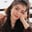Related Tags

pow
python
dataframe
pandas
communitycreator

# How to use the pandas DataFrame.pow() function in PythonFouzia Bashir

### Overview

In Python, the pandas DataFrame.pow() function is used to calculate the exponential power of DataFrame and other types of objects. The other type of objects can be sequence, series, constant, and more. It is equivalent to the DataFrame ** other operation.

Note: The ** operator can also be used, but it does not provide the facility to fill out empty fields, broadcast values across a level, and so on.

### Syntax

The syntax of the function is given below:

DataFrame.pow(other, axis='columns', level=None, fill_value=None)
Syntax of the DataFrame.pow() function

### Parameter values

The DataFrame.pow() function takes the following argument values:

• other: This can be DataFrame, sequence, series, or scalar.
• axis: defaut='columns', this can be 1, 'columns', 0, or 'index'.
• level: default=None, this broadcasts value across a level and can either be int or None.
• fill_value: default=None, this can either be a float value or None, and it helps to fill empty (NaN) fields.

### Return value

This method returns a DataFrame after performing certain arithmetic operationsCalculating power.

### Explanation

We can use this function in multiple ways. Some of the scenarios where we use this function are listed below:

• When both are DataFrames
• When there is a DataFrame and a constant
• When there is a DataFrame and series

### Both are DataFrames

Let's say we have two objects that are both DataFrames:

# importing pandas as pd
import pandas as pd
# Creating a the DataFrame
df1= pd.DataFrame({"A":[5, 9, 6, 4],
"B":[1, None, 4, 3],
"C":[4, 3, 8, None],
"D":[5, 4, 2, 7]})
# Creating another DataFrame
df2= pd.DataFrame({"A":[None, 4, 5, 9],
"B":[1, None, 4, 3],
"C":[14, 3, -1, None],
"D":[2, 4, 8, 8]})
# Evaluating floor division between df1 and df2
floorDiv= df1.pow(df2, fill_value = 3)
# print results
print(floorDiv)
• Lines 4–7: We create a DataFrame named df1, which has four rows and four columns.
• Lines 9–12: We create a DataFrame named df2, which has the same dimensions as df1.
• Line 14: We invoke the DataFrame.pow() function from the pandas module to calculate, element-wise, the exponential power between the DataFrames that we created.
• Line 16: We display the results on the console.

### DataFrame and constant

Let's say we have two objects, where one is a DataFrame and another is a constant:

# importing pandas as alias pd
import pandas as pd
# Creating a DataFrame
df = pd.DataFrame({"Alpha":[15, 3, 16, 8],
"Beta":[1, None, 4, 13],
"Charli":[4, 3, 8, None],
"Delta":[5, 1, 12, 8]})
# Invoking method for floor division
result= df.pow(4, fill_value = 5)
# print results on console
print(result)
• Lines 4-7: We create a DataFrame named df.
• Line 9: We invoke the df.pow() function to calculate, element-wise, the power 4 of the created DataFrame.
• Line 11: We print the resultant DataFrame on the console.

### DataFrame and series

Let's say we have two objects, where one is a DataFrame and another is a series:

# importing pandas as pd
import pandas as pd
import numpy as np
# Creating the DataFrame
df = pd.DataFrame([[15, 3, None, 4],
[11, None, 4, 3],
[4, 0, 8, None],
[5, 1, 2, 4]])
# Creating Numpy array
data = np.array([2, 1, 7, 9])
# Converting to Python series
series = pd.Series(data)
# Invoking floor division method
result= df.pow(series)
# printing results
print(result)
• Lines 5–8: We create a DataFrame named df, which consists of 16 numerical values that are distributed in a 4x4 frame.
• Line 12: We create a pandas's series that consists of four integer values.
• Line 14: We invoke the df.pow(series) function with series as an argument.
• Line 16: We print the results to the console.

RELATED TAGS

pow
python
dataframe
pandas
communitycreator

CONTRIBUTORFouzia Bashir
RELATED COURSES

View all Courses

Keep Exploring

Learn in-demand tech skills in half the time